# Support Vector Machines (SVM)

## Primal form

In the previous lab we have switched from the generative approach of classifier design to the discriminative one. Particularly, we have implemented the Perceptron algorithm. In this lab, we will continue examining the discriminative domain by implementing popular Support Vector Machine (SVM) classifier.

The perceptron algorithm has, despite its simplicity, several drawbacks. The first drawback is that it finds just some separating hyperplane (decision boundary), but ideally we would like to find the optimal separating hyperplane (at least in some sense). Another important drawback is, that perceptron cannot deal with noisy/non-separable data.

The SVM are designed to handle both of these drawbacks. Given an annotated training data set $$\mathcal{T} = \{ (\mathbf{x}_i, y_i)\}_{i = 1}^{m} \quad,\ \text{where} \ \mathbf{x}_i \in \mathbb{R}^n,\ y_i \in \{-1, 1\}$$ the SVM primal task is given as $$\mathbf{w^*}, b^*, \xi_i^* = \arg\min_{\mathbf{w}, b, \xi_i} \left( \frac{1}{2} \| \mathbf{w} \|^2 + C \sum_{i=1}^{m}{\xi_i} \right)$$ subject to

\begin{align} \langle\mathbf{w}, \mathbf{x}_i\rangle + b & \ge +1 - \xi_i, \quad y_i = +1, \\ \langle\mathbf{w}, \mathbf{x}_i\rangle + b & \le -1 + \xi_i, \quad y_i = -1, \\ \xi_i & \ge 0,\ \forall i. \end{align}

Here, and in the following text, $\langle\mathbf{a}, \mathbf{b}\rangle$ denotes dot product $\mathbf{a}^\top \mathbf{b}$. As we can see, the SVM is posed purely as an optimization task.

The first term in the above optimisation task maximises so called margin, $2/||\mathbf{w}||$, by actually minimising $||\mathbf{w}||$. In the separable case, the margin is the distance between the separating hyperplane and the closest training points (intuitively, the further is the separating hyperplane from the training data, the better it generalises to unseen data). Thus the SVM are sometimes referred to as Maximum margin classifier. More detailed explanation can be found in [1, Section 3 - Linear Support Vector Machines].

The sum in the minimisation task is over so called slack variables, $\xi_i$. They allow some training points to be incorrectly classified (compare the separating hyperplane equation in the optimisation constraints with the one in Perceptron) by paying certain penalty controlled by the user specified constant $C$. This enables SVM to deal with noisy data (linearly non-separable due to noise).

## Interactive demo

Do-it-yourself SVM optimisation. Try to get the SVM cost function as low as possible, while respecting the constraints.

1. Start by changing the hyper-plane parameters $w_1$, $w_2$ and $b$ (you can use UP and DOWN keys or mouse wheel) and slack variables. Constraint violation displayed in red.
2. Try adding more data points. Mouse click to add X-point (= class 1 training sample), shift+click to add O-point (=class 2 training sample), Ctrl+click to remove last point

DYI SVM demo. Try to get the SVM cost function as low as possible while respecting the constraints! Constraint violation displayed in red.
click: add X-point, shift+click: add O-point, ctrl+click: remove last point. (recommended way of interaction: click into an input field, use mouse scroll-wheel to change the value.)
$C$:

$\frac{1}{2} \| \mathbf{w} \|^2 + C \sum_{i=1}^{m}{\xi_i} =$
subject to:

$w_1$: $w$ angle:
$w_2$: $w$ length:
$b$:

## Dual form

Although intuitive, the primal formulation is difficult to optimize. Thus, the classifier parameters are usually sought by solving the dual form.

The derivation of the dual form can be found e.g. in  or . Note that to obtain the dual formulation, the student should be familiar with Lagrange multipliers.

The dual form is given as $$\mathbf{\alpha^*} = \arg\max_{\mathbf{\alpha}} \left( \sum_{i=1}^{m} \alpha_i - \frac{1}{2} \sum_{i=1}^{m}\sum_{j=1}^{m}\alpha_i y_i k(\mathbf{x}_i, \mathbf{x}_j) y_j \alpha_j \right),$$

subject to

\begin{align} C \ge \alpha_i & \ge 0,\quad i = 1, \dots, m \\ \sum_{i=1}^{m} \alpha_i y_i & = 0 \end{align}

Let us rewrite the dual task in matrix form:

$$\mathbf{\alpha^*} = \arg\max_{\mathbf{\alpha}} \left( \langle\mathbf{\alpha}, \mathbf{1}\rangle - \frac{1}{2} \mathbf{\alpha}^\top \mathbf{H} \mathbf{\alpha} \right),$$

subject to

\begin{align} \langle\mathbf{\alpha}, \mathbf{y}\rangle & = 0, \\ \mathbf{0} \le \mathbf{\alpha} & \le \mathbf{C} \end{align}

Having found the optimal vector of parameters $\mathbf{\alpha^*}$, a new data point $\mathbf{x'}$ is classified as

\begin{align} h(\mathbf{x}'; \mathbf{\alpha^*}, b^*) & = \text{sign}\left(\sum_{i=1}^{m} \alpha^*_i y_i k(\mathbf{x'}, \mathbf{x}_i) + b^*\right). \end{align}

Note that in the above summation, only the training samples where $\alpha_i > 0$ affect the classification. These training samples are called the support vectors, hence the name Support Vector Machine.

The calculation of the bias $b^*$ needed as the input for the above equation goes beyond the scope of this lab, and therefore we already provide a function compute_bias in the assignment template. Students who are more deeply interested in this problem are kindly referred to libSVM paper (Section 4.1.5) or an “easy to follow” explanation (exercise 4 solution).

If you are interested in more mathematical detail, check out this video from the MIT Artificial Intelligence course.

## Kernel function

In the dual form of the learning task as well as in the classification function all the data appear inside a dot product, so we used the so called kernel trick by introducing a function $k(\mathbf{x}, \mathbf{x}')$ which allows us to combine the dot product with a dimensionality lifting. This enables us to use a linear classifier (such as an SVM) on linearly non-separable data by increasing the dimensionality of the feature space.

Given a fixed feature space mapping $\phi(\mathbf{x})$, the kernel function is defined as follows $$k(\mathbf{x}, \mathbf{x}') = \phi(\mathbf{x})^\top\phi(\mathbf{x}')$$

As we can see, the kernel is a symmetric function of its arguments, i.e. $k(\mathbf{x}, \mathbf{x}') = k(\mathbf{x}', \mathbf{x})$. The simplest kernel is obtained when $\phi(\mathbf{x})$ is the identity mapping $\phi(\mathbf{x}) = \mathbf{x}$ resulting in the linear kernel $$k_L(\mathbf{x}, \mathbf{x}') = \mathbf{x}^\top\mathbf{x}'$$

The biggest advantage of using the kernel is that we do not even have to know the feature space mapping $\phi(\mathbf{x})$ explicitly. In fact it might even be a mapping to an infinite dimensional space! However not any symmetric function $k(x,x')$ is a kernel.

Here are some commonly used kernels you will need for the assignment:

• Linear kernel:
$k_L(\mathbf{x}, \mathbf{x}') = \mathbf{x}^\top\mathbf{x}'$

• Polynomial kernel of power $d$:
$k_P(\mathbf{x}, \mathbf{x}') = ( 1 + \mathbf{x}^\top\mathbf{x}' )^d$

• Gaussian kernel (aka RBF kernel):
$k_{RBF}(\mathbf{x}, \mathbf{x}') = e^{ \frac{ - \| \mathbf{x} - \mathbf{x}' \|^2_{2} }{2\sigma^2} }$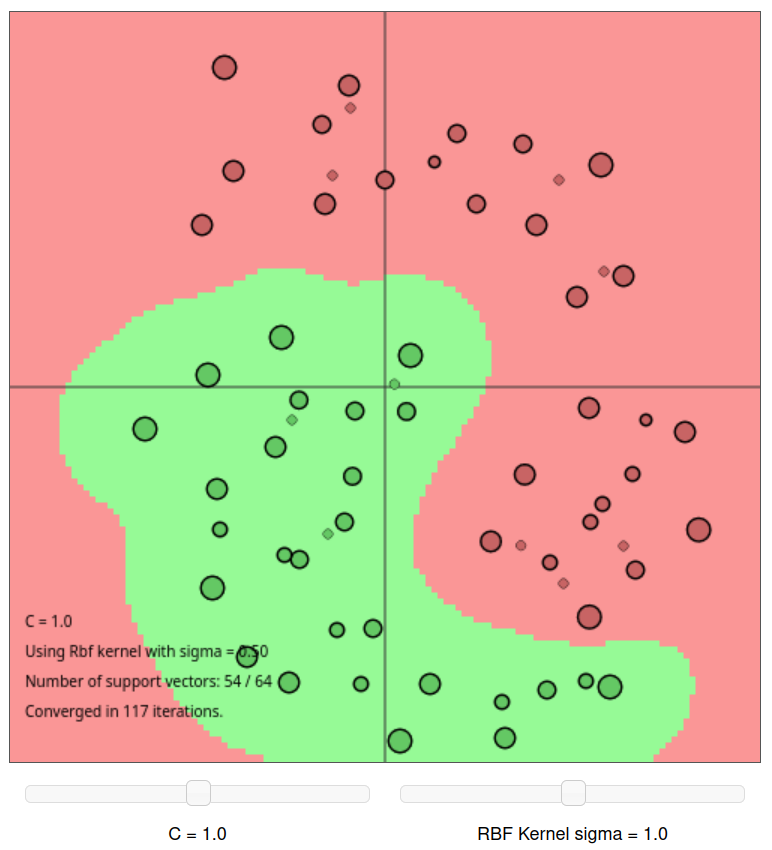Open the demo above (click on the image) and try to change the parameter C and the RBF kernel sigma to see how they influence the result.

## Part 1: Implement SVM training

To fulfil this assignment, you need to submit these files (all packed in a single .zip file) into the upload system:

• svm.ipynb - a jupyter notebook for data initialisation, calling of the implemented functions and plotting of their results (for your convenience, will not be checked)
• svm.py - containing the following implemented functions:
• get_kernel - a function implementing various kernels
• svm - a function which implements the learning of the kernel svm classifier
• classif_svm - a function which classifies given data
• svm_crossvalidation - a function computing mean cross-validation error
• compute_measurements_2d - a function which computes 2D features for given input data
• linear_svm.png, flower_rbf.png, flower_polynomial.png, ocr_polynomial_kernel_tst.png, ocr_svm_classif.png, mnist_tst_classif.png - images specified in the tasks

Use template of the assignment. When preparing a zip file for the upload system, do not include any directories, the files have to be in the zip file root.

1. Complete the function get_kernel(Xi, Xj, options).
Given the input matrices $\mathbf{X}_i$ of size $n \times p$ and $\mathbf{X}_j$ of size $n \times q$, the function outputs the kernel matrix ($p \times q$), where $n$ denotes dimensionality of the features and $p,\ q$ denote the number of examples. The type of a kernel is specified in field options[“kernel”].

Hint 1: See the template and the introductory text above for the definition of expected kernel implementations.
Hint 2: The reason why we allow different dimensions $p$ and $q$ is, because this allows us to easily use this function also in classification.

X = np.array([[1, 2, 1, -1, -1, -2], [1, 1, 2, -1, -2, -1]])
y = np.array([1, 1, 1, -1, -1, -1])

K = get_kernel(X, X, {'kernel': 'linear'})
print(K)
# -> [[ 2  3  3 -2 -3 -3]
# ->  [ 3  5  4 -3 -4 -5]
# ->  [ 3  4  5 -3 -5 -4]
# ->  [-2 -3 -3  2  3  3]
# ->  [-3 -4 -5  3  5  4]
# ->  [-3 -5 -4  3  4  5]]
K = get_kernel(X, X, {'kernel': 'polynomial', 'd': 2})
print(K)
# -> [[ 9 16 16  1  4  4]
# ->  [16 36 25  4  9 16]
# ->  [16 25 36  4 16  9]
# ->  [ 1  4  4  9 16 16]
# ->  [ 4  9 16 16 36 25]
# ->  [ 4 16  9 16 25 36]]
K = get_kernel(X, X, {'kernel': 'rbf', 'sigma': 1.0})
np.set_printoptions(precision=4, suppress=True)
print(K)
np.set_printoptions()
# ->  [[1.     0.6065 0.6065 0.0183 0.0015 0.0015]
# ->   [0.6065 1.     0.3679 0.0015 0.0001 0.    ]
# ->   [0.6065 0.3679 1.     0.0015 0.     0.0001]
# ->   [0.0183 0.0015 0.0015 1.     0.6065 0.6065]
# ->   [0.0015 0.0001 0.     0.6065 1.     0.3679]
# ->   [0.0015 0.     0.0001 0.6065 0.3679 1.    ]]

2. Complete the function svm(X, y, C, options). This function solves the dual task of the soft margin SVM and uses the kernel specified in options[“kernel”]. The output of this function is a python dict model which contains support vectors, labels of support vectors, non-zero Lagrange multipliers $\alpha$, dict options containing the information about the kernel used, the bias term in model[“b”] and the function which will be used for classification in model[“fun”] = classif_svm. For solving the quadratic program use the gsmo function included in the template.

Hint 1: Use the function get_kernel to compute the matrix $\mathbf{H}$ for QP task.
Hint 2: Use the provided compute_bias function.
Hint 3: For numerical reasons, it is more stable to compare $\alpha_i$ with a small non-zero constant (use $10^{-10}$) instead of zero when finding the support vectors.

X = np.array([[1, 2, 1, -1, -1, -2], [1, 1, 2, -1, -2, -1]])
y = np.array([-1, 1, 1, 1, -1, -1])
C = float('inf');
options = {'verb': True,
't_max': float('inf'),
'kernel': 'rbf',
'sigma': 0.02}

model = svm(X, y, C, options)
print(model)
# -> t=1, KKTviol=2.000000, tau=1.000000, tau_lb=0.000000, tau_ub=inf, Q_P=-1.000000
# -> t=2, KKTviol=2.000000, tau=1.000000, tau_lb=0.000000, tau_ub=inf, Q_P=-2.000000
# -> t=3, KKTviol=2.000000, tau=1.000000, tau_lb=0.000000, tau_ub=inf, Q_P=-3.000000
# -> {'sv': array([[ 1,  2,  1, -1, -1, -2],
# ->               [ 1,  1,  2, -1, -2, -1]]),
# ->  'y': array([-1,  1,  1,  1, -1, -1]),
# ->  'alpha': array([1., 1., 1., 1., 1., 1.]),
# ->  'options': {'verb': True,
# ->              't_max': inf,
# ->              'kernel': 'rbf',
# ->              'sigma': 0.01},
# ->  'b': 0.0,
# ->  'fun': <function classif_svm at 0x7fd4f1c56e18>}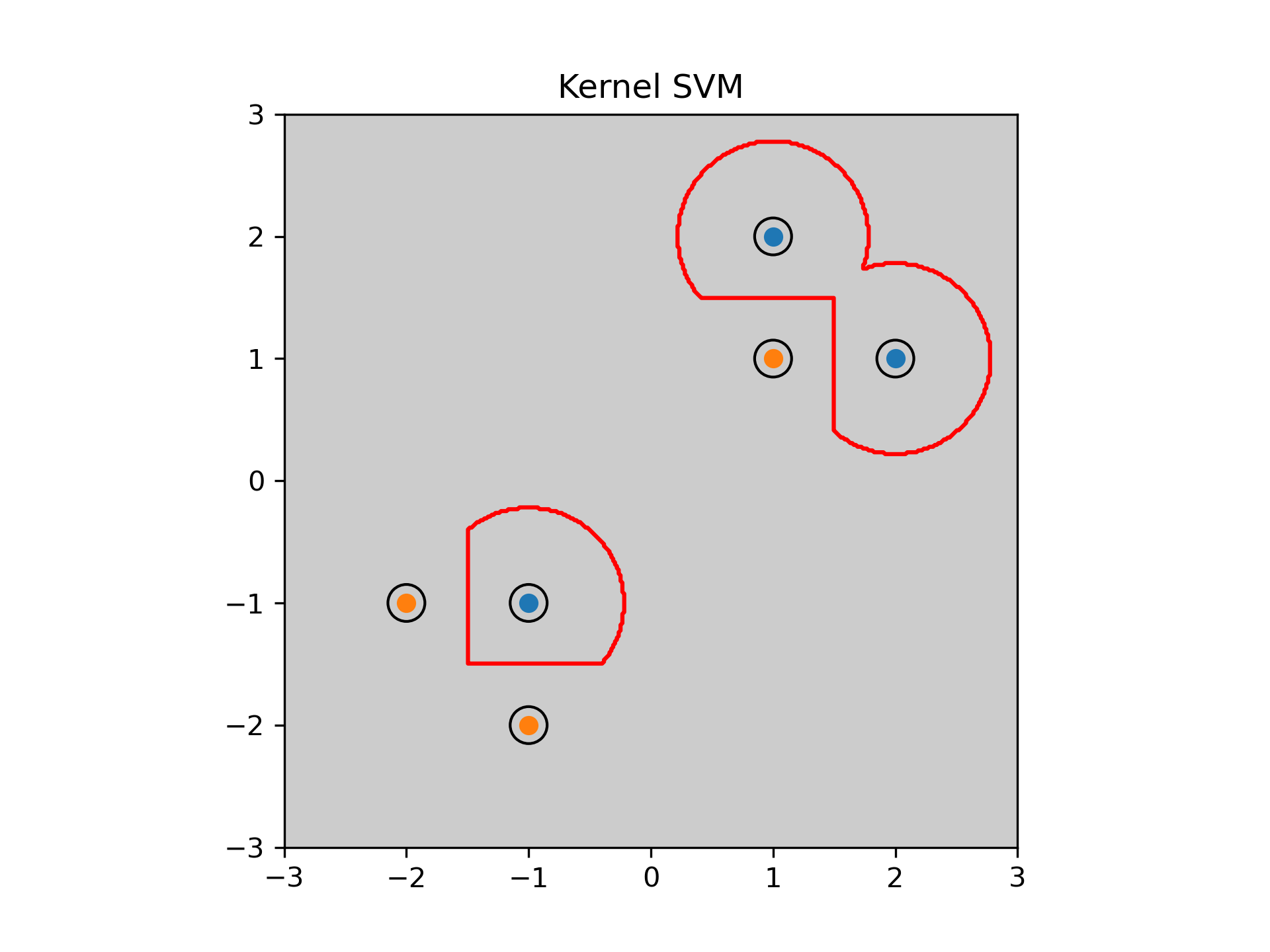3. Complete the function classif_svm(X, model).
The function applies a trained SVM classifier stored in model on the data X and outputs their classification labels.
This function is important for drawing the separating hyperplane by plot_boundary (provided in the template) and it will be used for classification of test data.

Hint 1: Recall that $f(\mathbf{x}) = \sum_{i=1}^r \alpha_i y_i k(\mathbf{x}_i, \mathbf{x}) + b$, where $\mathbf{x}_i$ denotes support vectors and $r$ is the number of support vectors.
Hint 2: Use function get_kernel to compute values of $k(\mathbf{x}_i, \mathbf{x})$ in $f(\mathbf{x})$.

# X and model are the same as defined above
classif = classif_svm(X, model)
print(classif)
# -> [-1.  1.  1.  1. -1. -1.]

## Part 2: Compare different kernels

1. Try out function svm with linear kernel on toy dataset (data_33rpz_svm_toy.npz) and experiment with the values of parameter $C$.
Hint: You can limit maximal number of iterations for gsmo algorithm by passing tmax. Default value is inf (which might for linearly non-separable data cause infinite loop for certain values of $C$).

X = np.array([[1, 2, 1, -1, -1, -2], [1, 1, 2, -1, -2, -1]])
y = np.array([1, 1, 1, -1, -1, -1])
C = np.inf;

model = svm(X, y, C, {'kernel': 'linear'})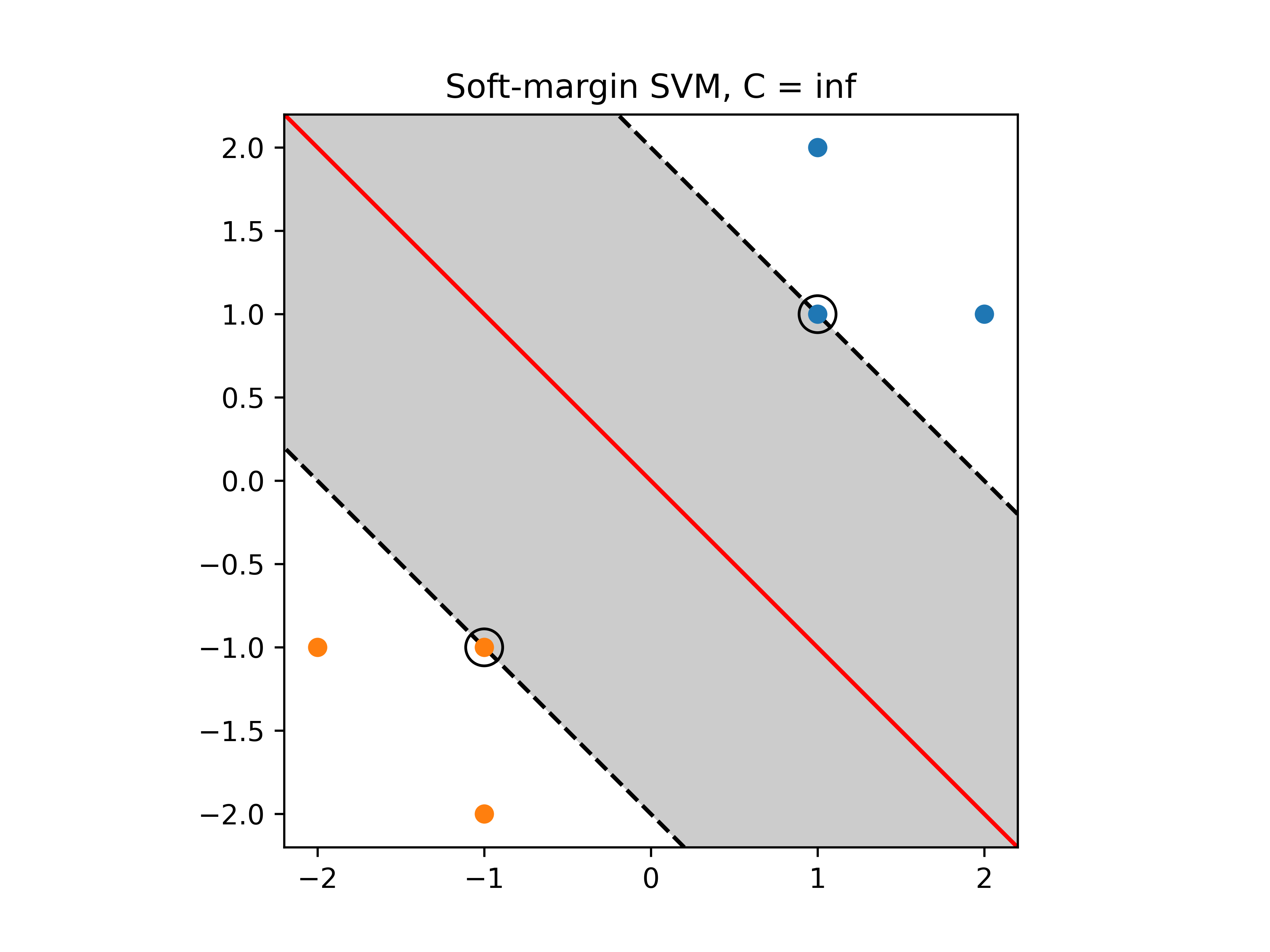2. Set the value of $C$ such that the resulting classifier has zero error on the training dataset (i.e. the data which you have used for training the SVM - data_33rpz_svm_toy.npz).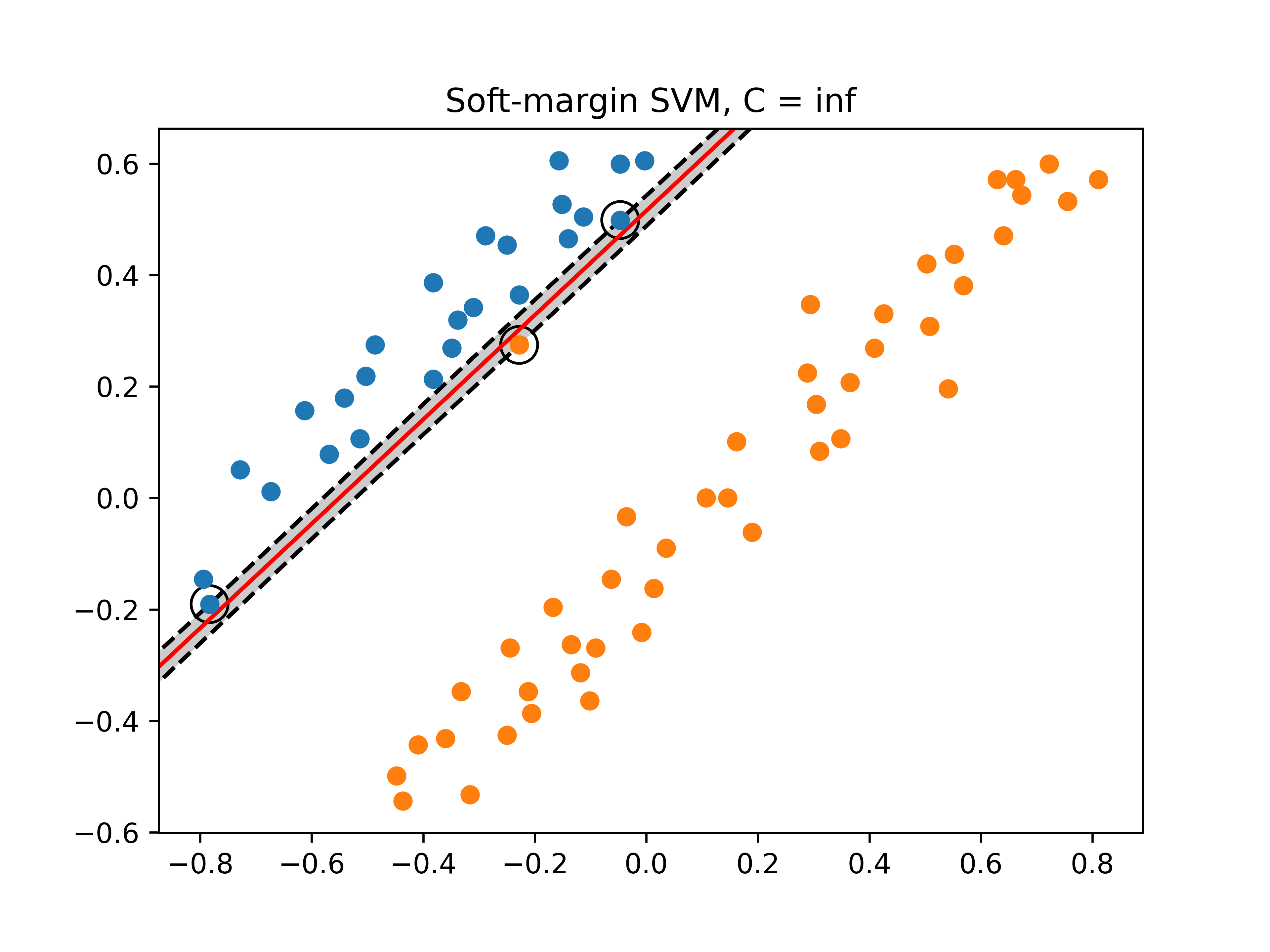Hint 1: You can use function classif_svm and compare its output with the vector $y$ used for training the SVM.
Hint 2: For plotting you can use the functions plot_points and plot_boundary provided in the template.
3. Plot results for $C = 1$ and highlight the support vectors. Save the figure linear_svm.png and submit it with your solution.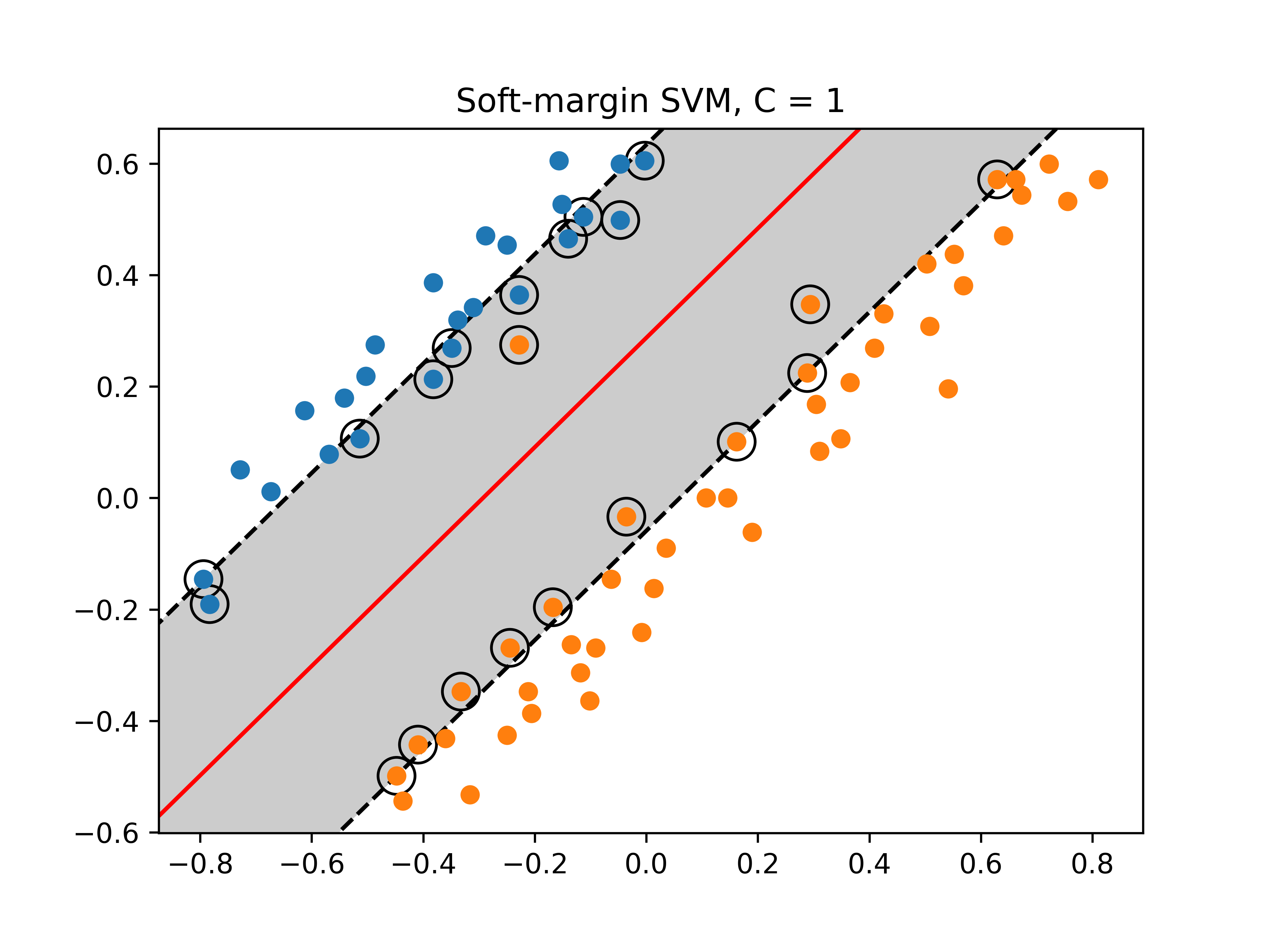4. Experiment with SVM on toy data flower.npz. Try to examine the effect of different kernels and their parameters.

5. Plot the decision boundaries along with the training data for the RBF and polynomial kernels. Save the figures into flower_rbf.png and flower_polynomial.png.

Hint: Use $C=10$ for RBF kernel and $C=10000$ for the polynomial kernel.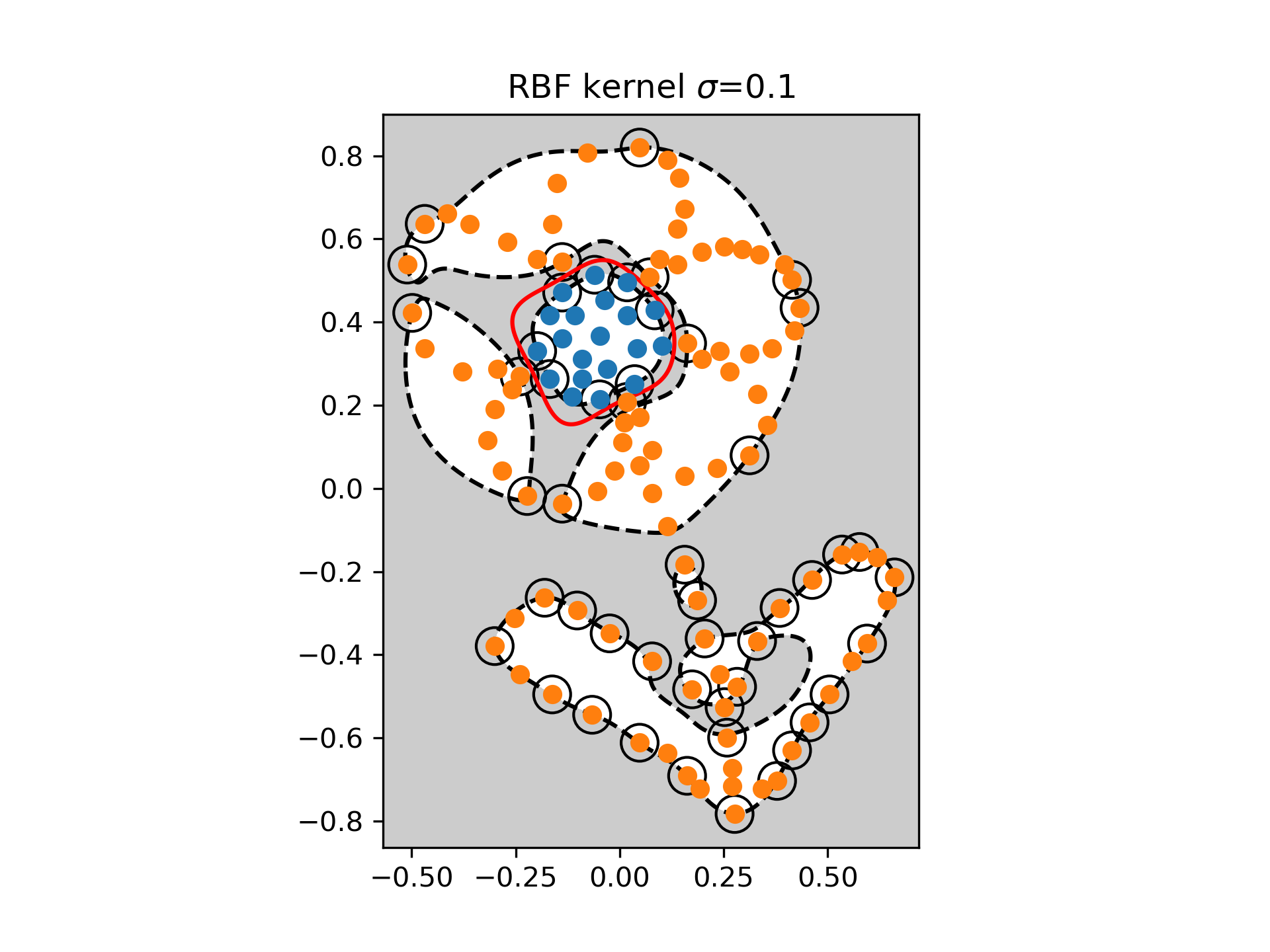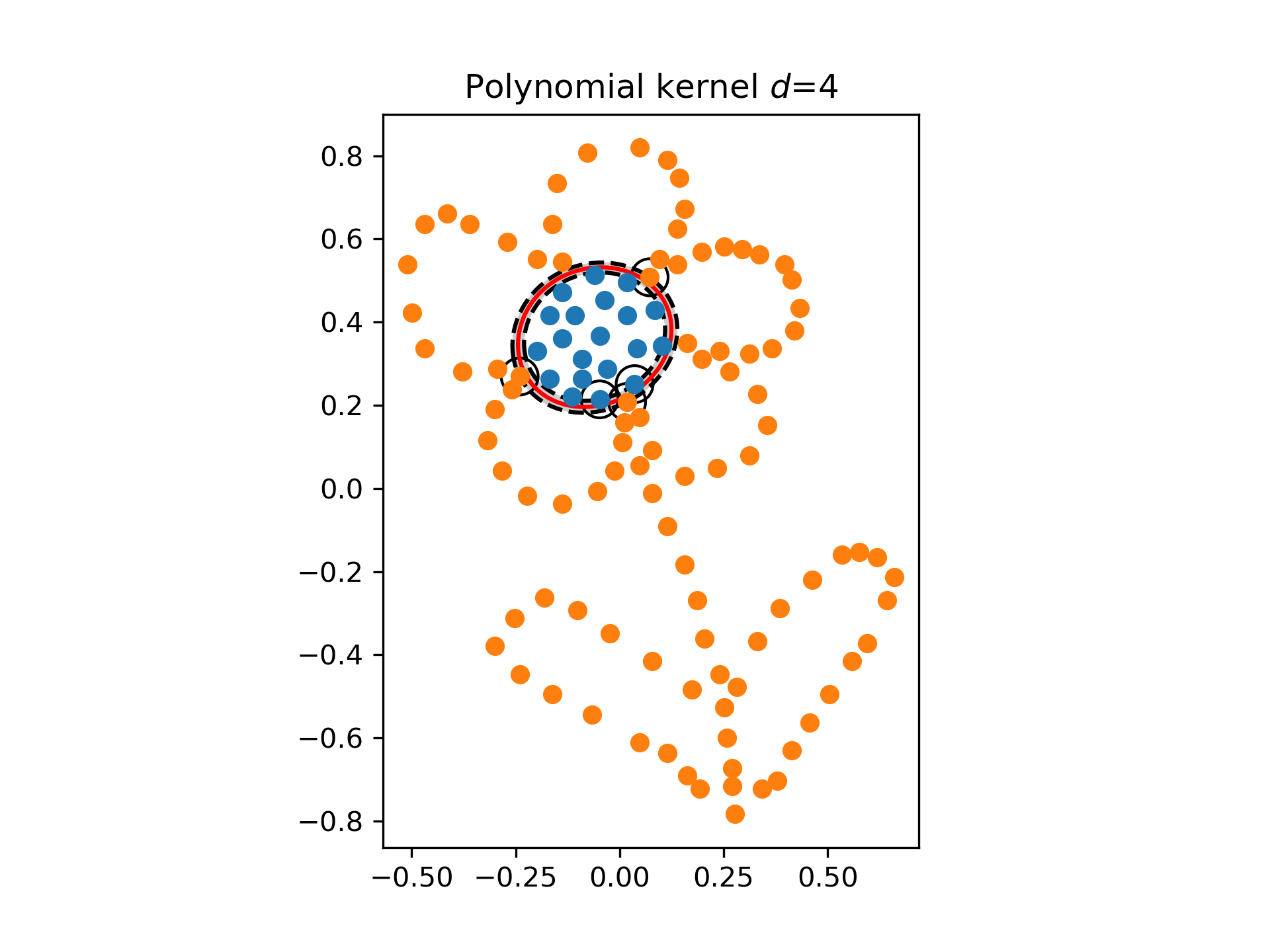## Part 3: Optimal parameter selection

Similarly to the Parzen Window task, we will use cross-validation to obtain optimal hyper-parameter values. In contrast to the previous task however, there are two hyper-parameters to be tuned - the misclassification penalty factor $C$ and the hyper-parameter of the kernel itself ($\sigma$ of the RBF kernel or $d$ of the polynomial kernel). Therefore, we will implement a 2D cross-validation.

In this part:

1. Prepare the OCR data for SVM training:
• Complete the template function compute_measurements_2d(data).
The function computes 2D features from the images, and you need to fill in the necessary normalization (it is needed for gsmo to converge). The output of this function is
• $X$ is a matrix $2 \times m$, $X = [\mathbf{a}; \mathbf{b}]$, $\mathbf{a} = [a_1, \dots, a_m]$, $\mathbf{b} = [b_1, \dots, b_m]$
• $a_i =$ (sum of pixel intensities in the left half of the image i) - (sum of pixel intensities in the right half of the image i)
• $b_i =$ (sum of pixel intensities in the top half of the image i) - (sum of pixel intensities in the bottom half of the image i)
• Normalize both $\mathbf{a},\ \mathbf{b}$ as follows:
$\mathbf{c}_{\text{norm}} = \frac{\mathbf{c} - \mathbf{\bar{c}}}{\sigma_{\mathbf{c}}}, \quad c \in \{\mathbf{a}, \mathbf{b} \},$
where $\mathbf{\bar{c}}$ denotes the average of $\mathbf{c}$ and $\sigma_{\mathbf{c}}$ denotes the standard deviation of $\mathbf{c}$. Normalization of data is very important. The normalization which we use here is referred to as standardization and is commonly used in machine learning. Note that by this normalization, the feature points are transformed in such way, that they have zero mean and unit variance. When classifying new data you should first standardize them with the same $\mathbf{\bar{c}}$ and $\sigma_{\mathbf{c}}$.
• $y$ is a (m, ) np.array with values $\{-1, 1\}$
Hint: Labels that originally had the value 2 will now have value -1.
Hint: Carefully work with datatypes. Input images are stored as uint8 (numpy array). It may cause unwanted behaviour within the sum.

2. Complete the function svm_crossvalidation(itrn, itst, X, y, C, options).
The function trains an SVM classifier on $X(\text{itrn{i}})$ and computes the error - the fraction of miss-classifications, $\text{Err(i)}$ on $X(\text{itst{i}})$.

Hint: Use functions svm and classif_svm.

3. Estimate the optimal values of parameters $C$ and $d$ (the degree of the polynomial kernel) using the features generated above
• Split the training set into 4 subsets using the crossval function (the one from the template).

• Use the function svm_crossvalidation to perform a 2D cross-validation for $C \in \{0.001, 0.1, 1, 10\}$ and $d \in \{1, 3, 5\}$.

Hint 1: Use two cycles, first one over $C$ and the second one over $d$, store the obtained mean test errors and find their minimum and corresponding parameter values.

4. Train an SVM with a polynomial kernel on the whole training dataset with the optimal parameters $C^*$ and $d^*$.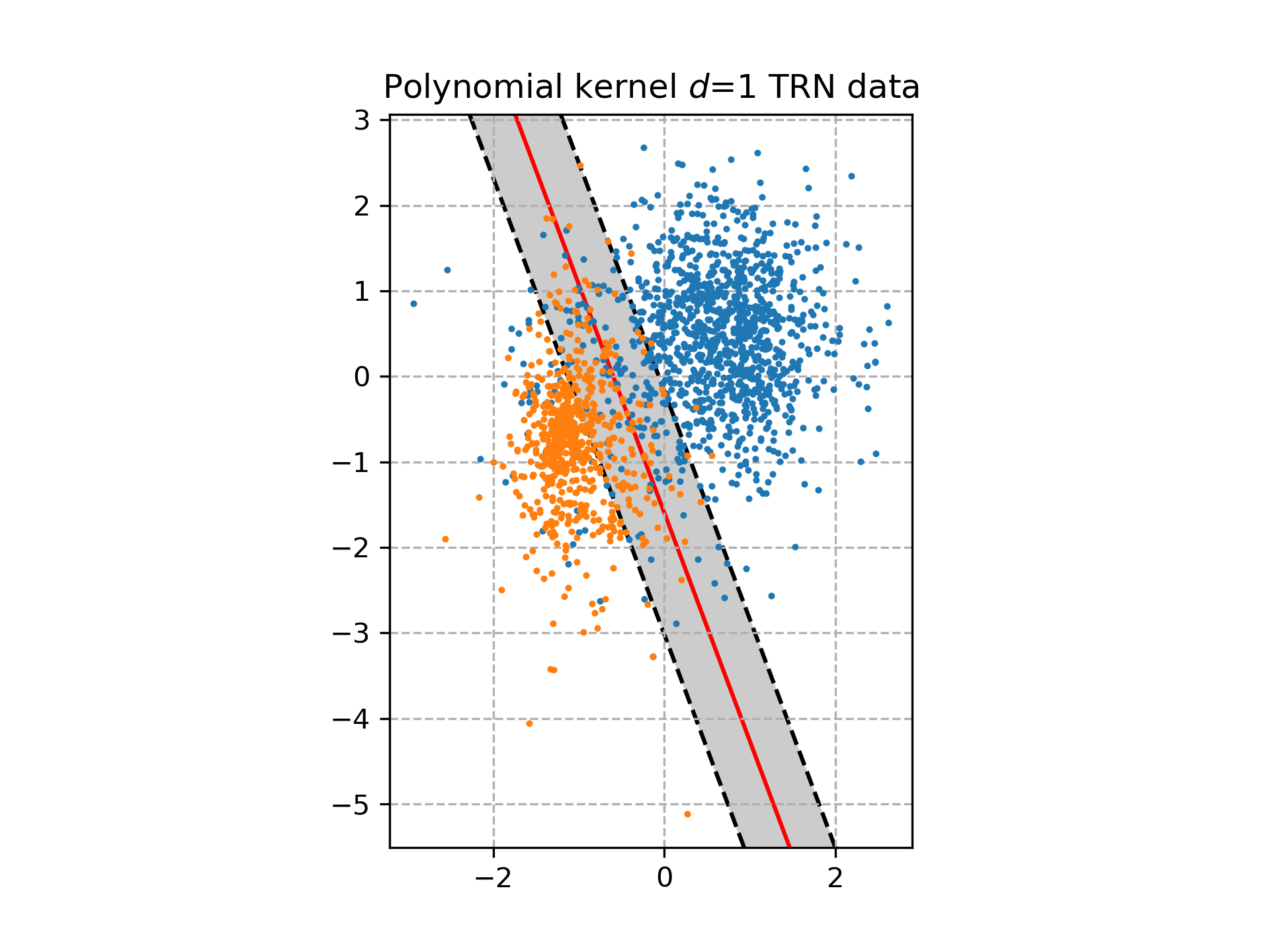5. Evaluate the trained SVM on the test data:

## Part 4: Real world example - digit classification

Finally, we will apply all the above methods to a real world example similar to what you may encounter in typical pattern recognition problems. We will use the MNIST database of hand-written numerals and will train an SVM classifier with RBF kernel for two numerals 0 and 1. In this case the dimensionality of features is much higher as we are going to use the pixel intensities directly (i.e. we have 784-dimensional measurements). Thus we cannot really plot the separating hyperplane, but we can still learn the SVM, do the cross-validation and classify.

Note that we have already normalized the data for you such that examples have zero mean and unit variance. It is also important to mention here, that the pixel intensities are not the best features we could use. Better results could be obtained with more sophisticated features e.g. the image gradients, local binary patterns or histogram of oriented gradients or some combinations of multiple features together. We refer to Feature detection as a starting point for those who would like a deeper understanding of the feature acquisition topic.

We have also limited the training set just to a relatively small number of examples, while for testing we have much bigger set. This is, of course, not typical as we should use as many training examples as possible. The reason for this is purely educative to ease the computational expenses as we do not expect that you have access to some grid computing system. In machine learning it is quite usual that it takes several hours (even up to days) to learn a classifier. Also note that while in our case learning could be very time consuming, the evaluation on test data is very fast.

1. Estimate the optimal values of the parameters $C$ and $\sigma$ (the width of the RBF kernel) on the MNIST training data mnist_trn.npz by using the 5-fold 2D cross-validation for $C \in \{0.01, 0.1, 1, 10\}$ and $\sigma \in \{0.1, 1, 10, 20, 100, 1000\}$.

2. Train an SVM with a RBF kernel on the whole training data with optimal parameters $C^*$ and $\sigma^*$.

3. Evaluate the trained SVM on the test data mnist_tst.npz:
1. Compute the classification error on the test data. You will need this value in the questions below.

2. Show the classification of the digits with the optimal classifier and save it as mnist_tst_classif.png

Hint: Use the function show_mnist_classification to display the classification.

Use MNIST data to create a multiclass classifier of images of numerals. The file contains 30 000 examples of normalized images of digits stored in matrix $X$ and corresponding labels (the label is the digit itself) $y \in \{0, 1, \dots, 9\}$. Use actual pixel values as features, in this case your feature space will be 784 dimensional.
Display the resulting classification (i.e. the montage of all images classified as $0, 1, \dots, 9$).